The Perception of Love from Machines
MAT 259, 2022
Siming, Su

Concept
For this final project, I would like to make a visualization that analyses the attractiveness of certain users in a dating app by using three machine learning algorithms, which are Linear Regression, XGBoost, and Neural Netowrk. The data is taken from Kaggle website. Kaggle DataSet There are 42 variables in this dataset. After data cleaning, 25 variables are selected. Then, I would use different Machine Learning Algorithms to see different perceptions on attractiveness from each algorithm by predicitng the variable "Counts_Kisses" (which is the like you get from other users).
I would explain why I use these three algorithms and the nice intuition behind these three machine learning algorithms.

Preliminary sketches
I decided to put each algorithm into each box, and make them look like a heart itself. Since predictions do not have 100 percent accuracy. Thus, I measure each algorithm by RMSE (sqaure root of mean squared Residuals), then let each box size represents accuracy rate. ie. If RMSE is smaller, accuracy will be larger, which means the box will be larger.rouch scratch of the project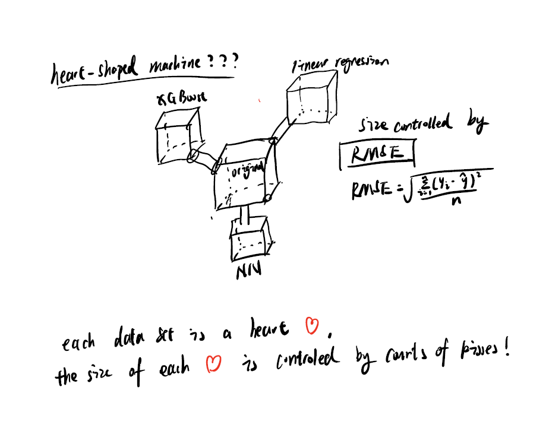Process
Linear Regression: Linear Regression is basically to fit a linear polynomial equation to the whole dataset by fitting coefficients and intercept.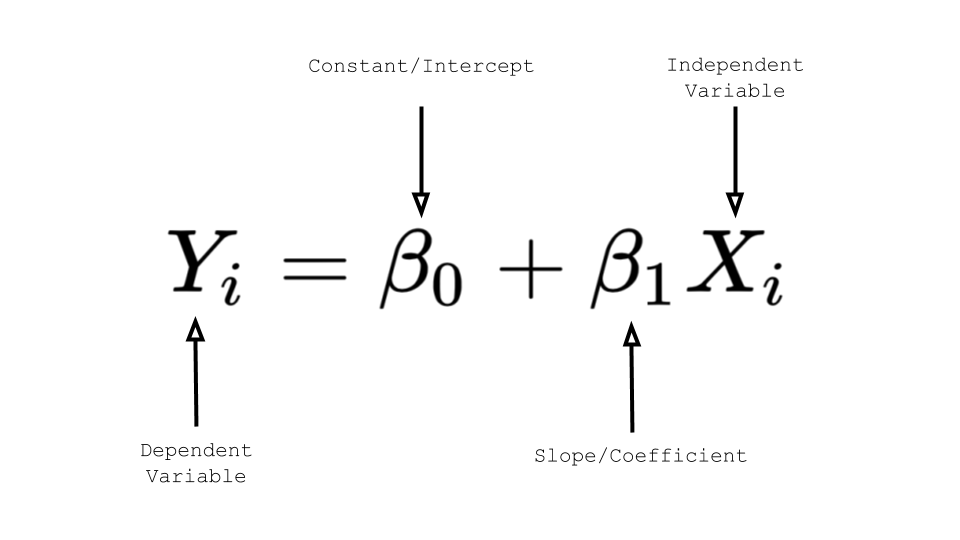In this case, $$Y_i$$ is the variable we would like to predict. $$\beta_0$$ is the intercept, and beta_1 is the coefficient. Right now, our fitted coefficient by R is the following.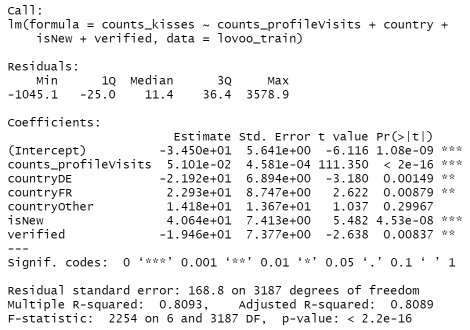Then, our formula will be the following: $${\hat{Y_i} =-34.5 + 0.05 x_1 * - 21.92 * x_2 + 22.93 * x_3 + 14.18 * x_4+ 40.64 * x_5 - 19.46 * x_6}$$ This means keep other variables fixed, if you get one more profile visit (x_1), then the like you get from other users will increase by 0.05. The same idea goes for x_2, the difference is that x_2 is a binary categorical dummy variable of {0: Not from Germany,1: from Germany}. Then intuition is that if the user is from Germany, Then, the like you get from other users will decrease for 21.92. x_3 is {0:Not from France, 1: from France}. x_4 is {0: Not from other country, 1: From other country}. x_5 is {0: old user, 1:new user}. x_6: {0:Not Verified, 1:Verified}.

Neural Network: Neural Network is an algorithm that use neuron nets to train the model by setting the corresponding neuron weight for each backpropogation (gradient descent by chain rule). If you would like to know more about this, check out the video right here.

In my project, I will visualize how a pre-trained neural network model perceives attractiveness of users on the dating app. Compared to Linear Regression, neural network is not that interpretable, but it is more accurate than Linear Regression in a sense that this model captures more than just linear relationship among variables.
XGBoost (Extreme Gradient Boosting): This algorithm is designed specifically to increase accuracy of data. Before getting to know XGBoost, we need to know decision tree first. Decision tree is an algorithm that uses spliting algorithm such as Gini Index or entropy loss to build a tree by spliting the nodes. Then, such a tree could be used to make predictions. The following result I got is from training my dataset.XGBoost is a ensemble method such that it uses multiple trees to train at the residuals by setting diferent weight each time. The following picture describes this process.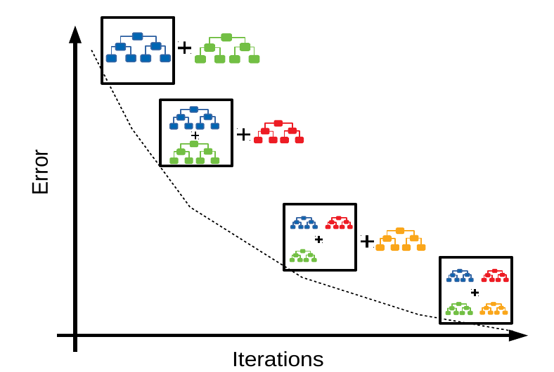This is a video of original idea about neural network. This is a rudimentary result, and I have improved it on my final version.

>

Final result
Video and pictures of final project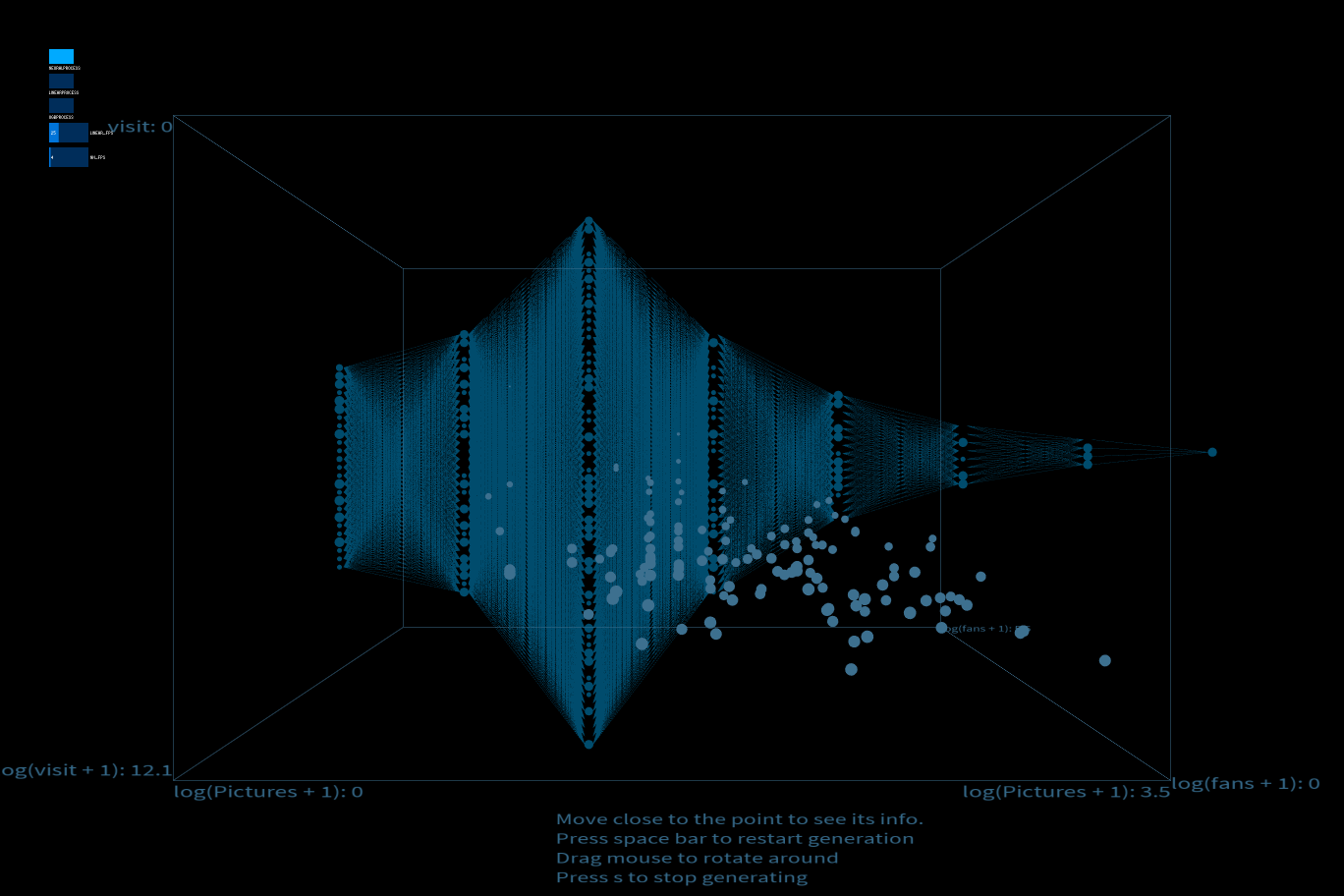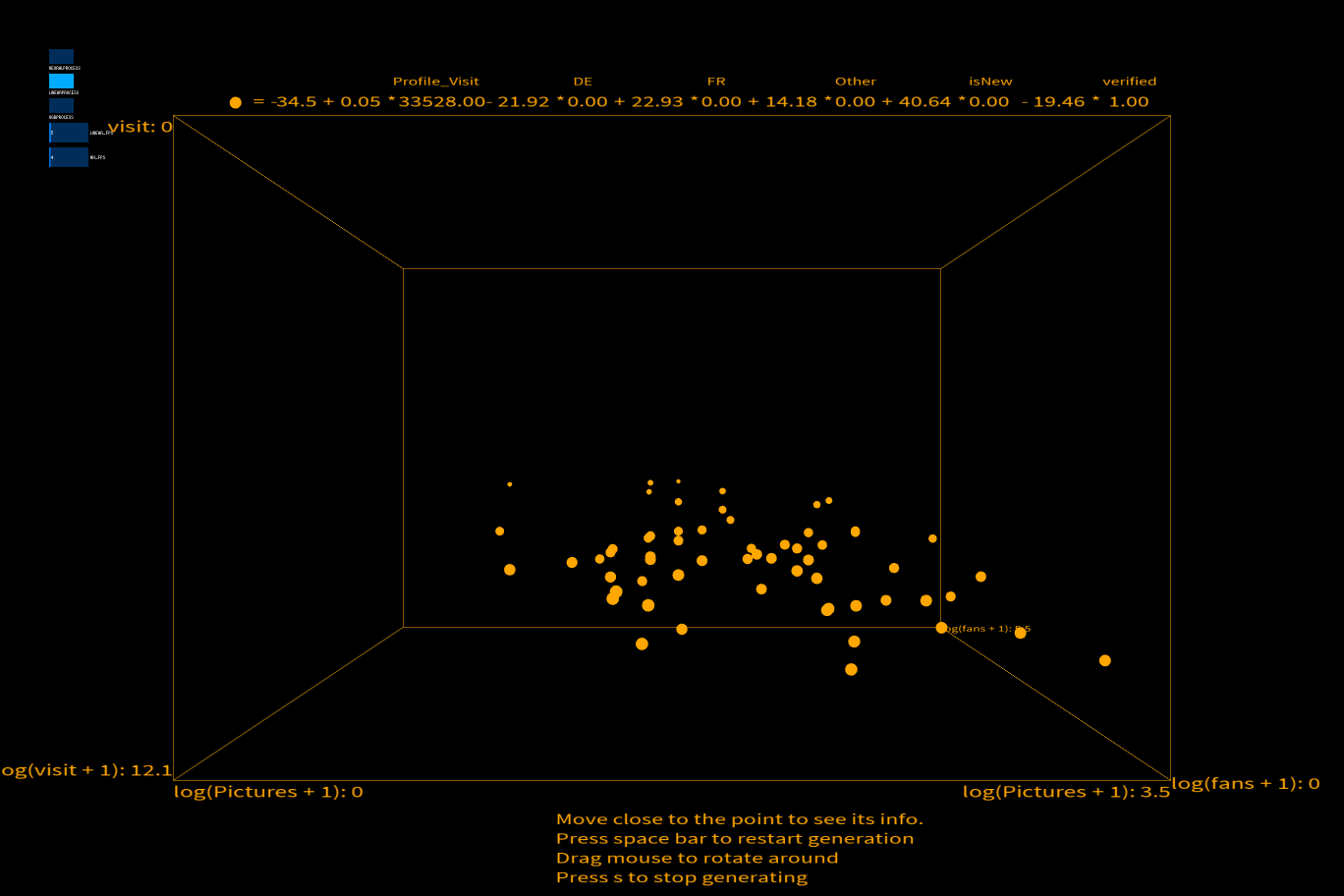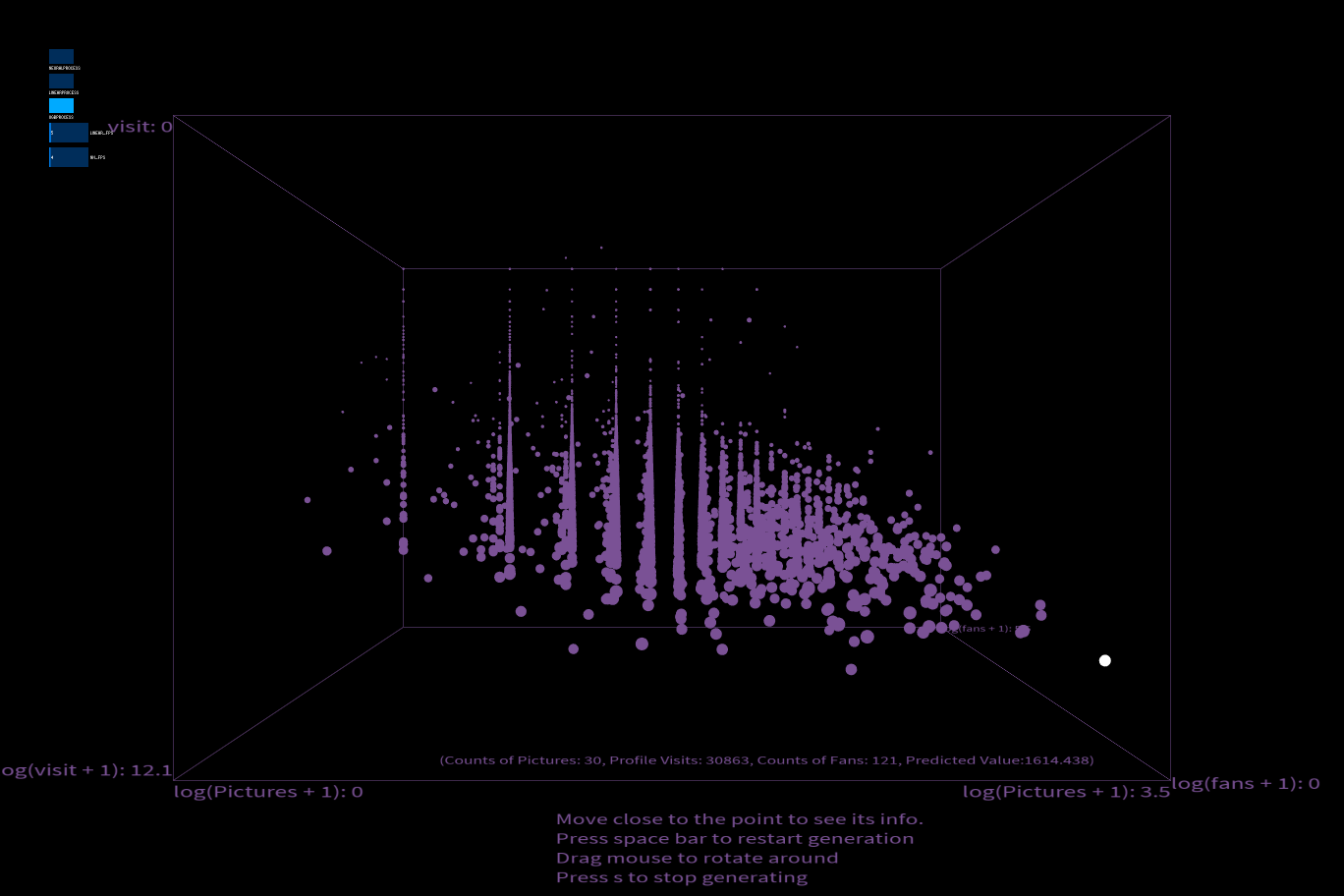Code
All work is developed within Processing and Python
Source Code + Data